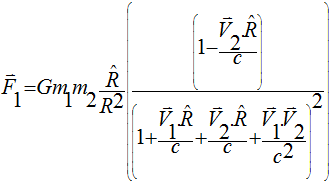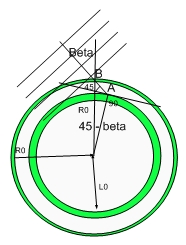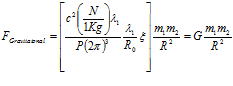# There is No Blinder Man (Than The One Who Refuses to See)..:)

## There is No Blinder Man (Than The One Who Refuses to See)...:)

As expected, obvious errors in our current Cosmological Model would become more and more evident and data piles up. Horrendous logical mistakes like the Inflation Theory will eventually be dropped like it is Hot..:)

The paper below summarizes the state of confusion...:)

Title: The Failures of the Standard Model of Cosmology Require a New Paradigm
Authors: Kroupa, Pavel; Pawlowski, Marcel; Milgrom, Mordehai

Cosmological models that invoke warm or cold dark matter cannot explain observed regularities in the properties of dwarf galaxies, their highly anisotropic spatial distributions, nor the correlation between observed mass discrepancies and acceleration. These problems with the standard model of cosmology have deep implications, in particular in combination with the observation that the data are excellently described by Modified Newtonian Dynamics (MOND). MOND is a classical dynamics theory which explains the mass discrepancies in galactic systems, and in the universe at large, without invoking 'dark' entities. MOND introduces a new universal constant of nature with the dimensions of acceleration, a0, such that the pre-MONDian dynamics is valid for accelerations a ≫ a0, and the deep MONDian regime is obtained for a ≪ a0, where spacetime scale invariance is invoked. Remaining challenges for MOND are (i) explaining fully the observed mass discrepancies in galaxy clusters, and (ii) the development of a relativistic theory of MOND that will satisfactorily account for cosmology. The universal constant a0 turns out to have an intriguing connection with cosmology: ā0 ≡ 2πa0 ≈ cH0 ≈ c2(Λ/3)1/2. This may point to a deep connection between cosmology and internal dynamics of local systems.

Needless to say, the MOND model is also nosensical.  It requires changing Newtons laws without providing any basis for it..:)

# Modified Newtonian dynamics

"MOND" redirects here. For other uses, see Mond.

In physics, modified Newtonian dynamics (MOND) is a theory that proposes a modification of Newton's laws to account for observed properties of galaxies. Created in 1983 by Israeli physicist Mordehai Milgrom, the theory's original motivation was to explain the fact that the velocities of stars in galaxies were observed to be larger than expected based on Newtonian mechanics. Milgrom noted that this discrepancy could be resolved if the gravitational force experienced by a star in the outer regions of a galaxy was proportional to the square of its centripetal acceleration (as opposed to the centripetal acceleration itself, as in Newton's Second Law), or alternatively if gravitational force came to vary inversely with radius (as opposed to the inverse square of the radius, as in Newton's Law of Gravity). In MOND, violation of Newton's Laws occurs at extremely small accelerations, characteristic of galaxies yet far below anything typically encountered in the Solar System or on Earth.

MOND is an example of a class of theories known as modified gravity, and is an alternative to the hypothesis that the dynamics of galaxies are determined by massive, invisible dark matter halos. Since Milgrom's original proposal, MOND has successfully predicted a variety of galactic phenomena that are difficult to understand from a dark matter perspective. However, MOND and its generalisations do not adequately account for observed properties of galaxy clusters, and no satisfactory cosmological model has been constructed from the theory

My theory derives Newton's Gravitational Law from FIRST PRINCIPLES and demonstrate that there is a velocity dependence on the Force or Gravitational Potentialthis means that as the center of the Galaxy rotates it provides and extra drag on the outer stars.  If the central Black Hole is also rotating, that force in itself will be enough to explain this unexplainable observation without the need for Hocus Pocus MOD...:)

The observed acceleration was deemed to have an "intriguing connection wit Cosmology". a0 ≈ cH0

From the simple picture below one can easily derive H0 for short distances:

### H0=c/R

where R is the Radius of the Universe along the Radial direction. Of course, since a0=cH0

One easily obtain a0=c2/R, which is the centripetal acceleration, resulting from the fact that one is using light to measure distance on a non-inertial curve framework, which we are considering to be flat.

So the relationship is "intriguing indeed"...   :) but also OBVIOUS if you consider the Hypergeometrical Universe Model.In a reasonable Universe, I would just call the fellow and suggest this simple model.  In this Universe, the Blinder Scientists will bump into data and somehow miss the obvious solution to the problem until one of them achieves Genius Status, grow a pair and rediscover my model...:)

Well, I ended up sening Marcel Pawlowski an email.  Waiting from a reply.

Cheers,

MP

PS_  The anisotropy associated with observations should be easily understood if you remember my gyrogravity equation and its derivation.The Inflation Model (together with the Higgs Boson) can be easily rejected as the largest example of Herd Behavior in modern times. I was going to be more definite but then I remembered the Dark Ages.  Scientists at the time were quite obedient and accepting of Religious Views..(if for no other reason, just to avoid being killed by those nice Religious Folks...:)

This equation displays how the Gravitational constant scales with R0, the radius of the Universe. The Radius of the Universe is c time the Age of The Universe, so when viewing very far away Stellar Candles, you should take into consideration that Gravitation was stronger (in relation to electromagnetism) and thus the equilibrium between Gravitation and Thermonuclear Energy was displaced towards smaller masses.  Smaller stellar masses means weaker candles, thus overestimated values for their distances (Stellar Candles are suppose to have constant peak luminosity, so if their peak luminosity changes with distance, their calculated distances will be incorrect - this is the reason why Guth created the Inflation Theory - giving Inflation a bad name).Currently unrated

### New Comment

required

required (not published)

optional

required